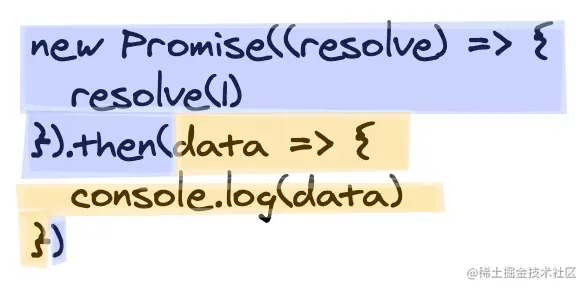# Promise 你真的用明白了么？

Promise 关于 API 这块大家应该都能熟练使用，但是和微任务相关的你可能还存在知识盲区。

## 前置知识

### Promise 哪些 API 涉及了微任务？

Promise 中只有涉及到状态变更后才需要被执行的回调才算是微任务，比如说 `then``catch``finally` ，其他所有的代码执行都是宏任务（同步执行）。### 这些微任务何时被加入微任务队列？

• 如果此时 Promise 状态为 pending，那么成功或失败的回调会分别被加入至 `[[PromiseFulfillReactions]]``[[PromiseRejectReactions]]` 中。如果你看过手写 Promise 的代码的话，应该能发现有两个数组存储这些回调函数。

• 如果此时 Promise 状态为非 pending 时，回调会成为 Promise Jobs，也就是微任务。

## 初级

``````Promise.resolve()
.then(() => {
console.log("then1");
Promise.resolve().then(() => {
console.log("then1-1");
});
})
.then(() => {
console.log("then2");
});
``````

## 中级

``````let p = Promise.resolve();

p.then(() => {
console.log("then1");
Promise.resolve().then(() => {
console.log("then1-1");
});
}).then(() => {
console.log("then1-2");
});

p.then(() => {
console.log("then2");
});
``````

``````let p = Promise.resolve().then(() => {
console.log("then1");
Promise.resolve().then(() => {
console.log("then1-1");
});
}).then(() => {
console.log("then2");
});

p.then(() => {
console.log("then3");
});
``````

## 高级

``````Promise.resolve()
.then(() => {
console.log("then1");
Promise.resolve()
.then(() => {
console.log("then1-1");
return 1;
})
.then(() => {
console.log("then1-2");
});
})
.then(() => {
console.log("then2");
})
.then(() => {
console.log("then3");
})
.then(() => {
console.log("then4");
});
``````

• 第一次 `resolve` 后第一个 `then` 的回调进入微任务队列并执行，打印 `then1`

• 第二次 `resolve` 后内部第一个 `then` 的回调进入微任务队列，此时外部第一个 `then` 的回调全部执行完毕，需要将外部的第二个 `then` 回调也插入微任务队列。

• 执行微任务，打印 `then1-1``then2`，然后分别再将之后 `then` 中的回调插入微任务队列

• 执行微任务，打印 `then1-2``then3` ，之后的内容就不一一说明了

``````Promise.resolve()
.then(() => {
console.log("then1");
Promise.resolve()
.then(() => {
console.log("then1-1");
return Promise.resolve();
})
.then(() => {
console.log("then1-2");
});
})
.then(() => {
console.log("then2");
})
.then(() => {
console.log("then3");
})
.then(() => {
console.log("then4");
});
``````

PS：`then`** 返回一个新的 Promise，并且会用这个 Promise 去 `resolve` 返回值，这个概念需要大家先了解一下。**

### 根据 Promise A+ 规范

``````if (x instanceof MyPromise) {
if (x.currentState === PENDING) {
} else {
x.then(resolve, reject);
}
return;
}
``````

### 根据 ECMA - 262 规范

This Job uses the supplied thenable and its then method to resolve the given promise. This process must take place as a Job to ensure that the evaluation of the then method occurs after evaluation of any surrounding code has completed.